2001400×440×210550×120×750657×120×750700×440×210600×570×900Φ264660×25×914900×600×898600×570×915750×116×8001500×850×139243×140×750508×120×762600×550×870750×570×915914×19×914610×19×914730×220×7151200×600×898449×215×63489×116×762590×28×800600×116×800664×95×724760×600×118914×22×914定制1000×8001200×610×918610×22×914747×142×633800×130×1027914×25×9141000×130×11001200×850×6001500×531×7751900×900×20386×762×116440×700×125450×700×140500×117×815500×133×762590×125×762590×133×762595×570×875600×140×700600×145×725600×800700×198×800742×570×875750×128×700750×140×700750×31×900750×570×870750×570×885751×570×864753×570×864762×19×914885×449×430885×449×780900×154×785900×850×121980×50×7001000×36×10001080×130×9351100×700×231340×36×1000150×120×2102000×610×918300×133×762354×208×180450×121×570489×129×762489×762503×133×775503×775508×123.8×852550×135×700580×120×720600×120×680600×800×20600×900×118610×133×762660×700×125680×115×950700×18×900700×900×20740×540×638750×135×700750×22×1000760×132×970800×130×935800×185×950800×500×850850×135×700900×115×950900×132×1020900×381×122900×545×790930×29×1000950×22×1000950×30×714Φ200Φ9501000×150×8001000×155×7501000×165×7101000×167×9201000×31×10001000×580×1801025×210×6651030×210×6651080×145×6801100×160×8001100×170×10001100×50×7001170×130×6001180×600×351185×449×4301185×449×7801200×130×6201200×145×7251200×154×7851200×165×7501200×50×7001200×550×8491200×85×8101350×225×6651400×23×7301600×130×620160×700180×120×353213×2652300×1200250×140×750265×213350×200×800381×122×662386×116×797420×97×307480×700500×160×800500×627×18500×800×5500×850508×122×662508×122×760508×122×805508×124×788508×136×752510×135×662530×155×750535×33×733540×23×900550×381×122550×880×15555×118×748572×140×772576×152×790580×50×2150590×116×797590×125× 762590×158×776590×762595×142×633600×112×760600×133×762600×160×800600×23×800600×480×52600×750600×800×118609×116×780629×13×844635×122×662640×148×850650×820×120700×115×720700×120×856700×136×805700×140×720700×160×800700×45×800700×50700×900705×30750×145×725750×165×750750×20×900750×381×122750×570×210750×570×864752×570×864762×122×662762×95×18770×50×815772×140×772775×585×848790×135×800800×1000800×125×700800×140×500800×160×800800×500×5810×810820×130×1040850×50×600860×135×870866×120×762866×133×762890×122×664890×595×50900×100×650900×115×680900×130×1000900×145×725900×160×800900×165×750900×198×800900×36×700900×45×800900×481×52900×550×800900×600900×800910×140×770972×140×772Φ1200φ550×45φ750×451000×120×6101000×120×7101000×120×8001000×130×5001000×135×6501000×145×8001000×175×8501000×20×5001000×30×6501000×35×7001000×37×5501000×50×5001000×520×8301000×520×8501000×5501000×560×201000×6001000×600×51000×750×51000×800×51000×850×130100×850×301030×915×5951045×45×10101050×735×1501050×760×301060×135×4701090×150×6801090×436×7501100×150×6901100×20×5501100×60×1301100×930×351104×910×631114×150×9801116×37×10101117×1131×801140×35×9551143×159×11731150×700×1381150×700×1401160×145×8001160×60×11501170×138×7771180×150×8001194×142×6331194×167×7251200×100×5601200×110×7501200×115×7371200×11651200×120×8001200×140×4001200×167×9201200×186×8001200×4501200×45×10201200×50×6001200×50×6801230×175×8501270×1270×671300×480×9101300×58×8901320×155×7951320×500×7601350×45×10821350×500×7501370×500×990

20

2020201920182017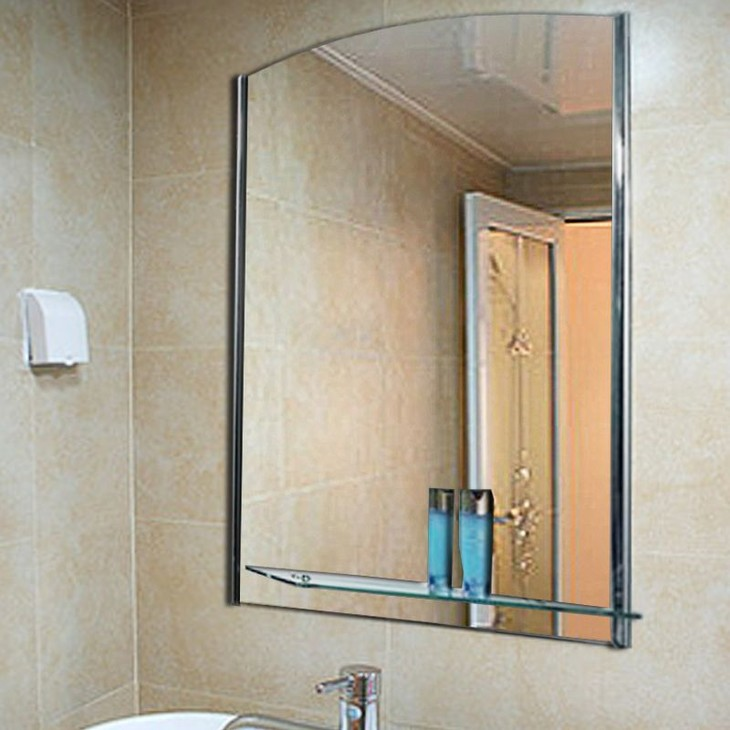13%
2020/09/2713%
2020/10/18

13%
2020/09/2513%
2020/10/18

13%
2020/09/2513%
2020/10/18

13%
2020/09/2513%
2020/10/18

13%
2020/09/2513%
2020/10/18

13%
2020/09/2513%
2020/10/18

13%
2020/09/2513%
2020/10/18

13%
2020/09/2513%
2020/10/18

13%
2020/09/2513%
2020/10/18

13%
2020/09/2513%
2020/10/18

13%
2020/09/2513%
2020/10/18

13%
2020/09/2513%
2020/10/18

13%
2020/09/2513%
2020/10/18

13%
2020/09/2513%
2020/10/18

13%
2020/09/2513%
2020/10/18

13%
2020/09/2513%
2020/10/18

13%
2020/09/2513%
2020/10/18

13%
2020/09/2513%
2020/10/18

13%
2020/09/2513%
2020/10/18

13%
2020/09/25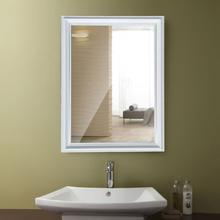13%
2020/10/18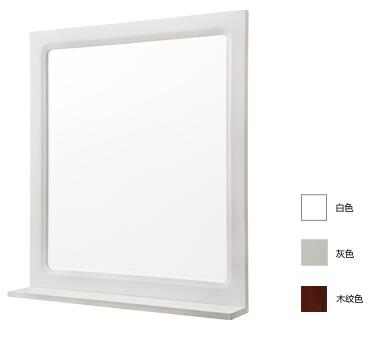13%
2020/10/1813%
2020/10/18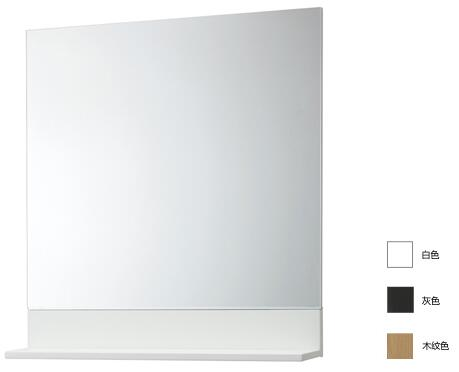13%
2020/10/18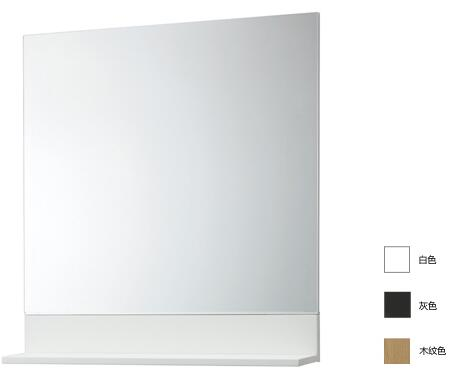13%
2020/10/1813%
2020/10/1813%
2020/10/18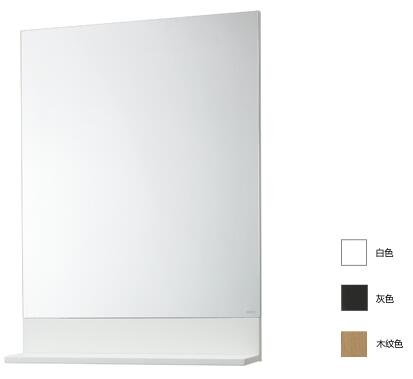13%
2020/10/1813%
2020/10/1813%
2020/10/18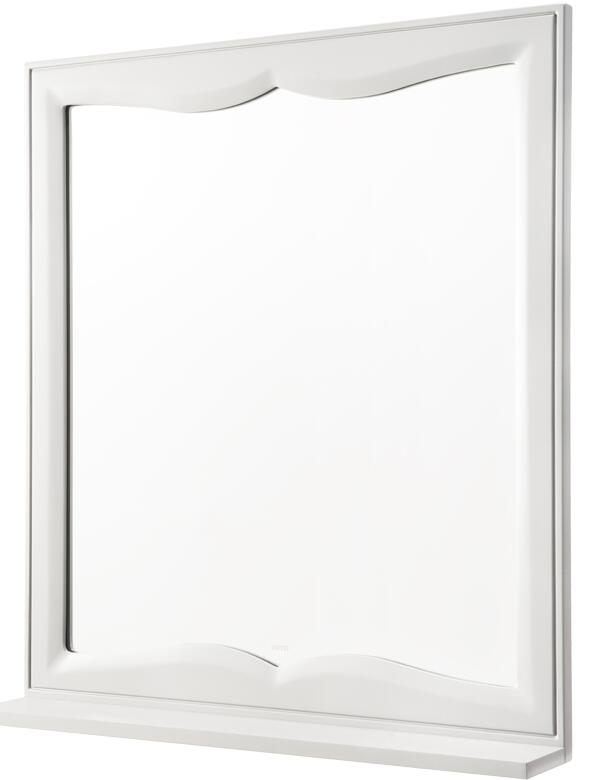13%
2020/10/18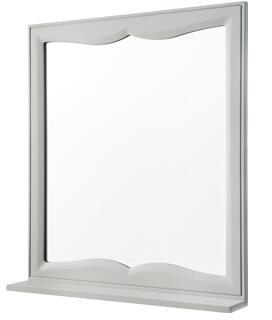13%
2020/10/1813%
2020/10/18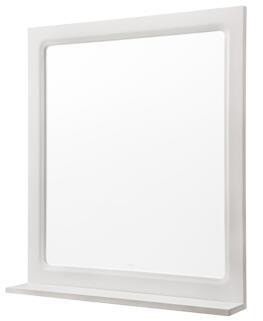13%
2020/10/18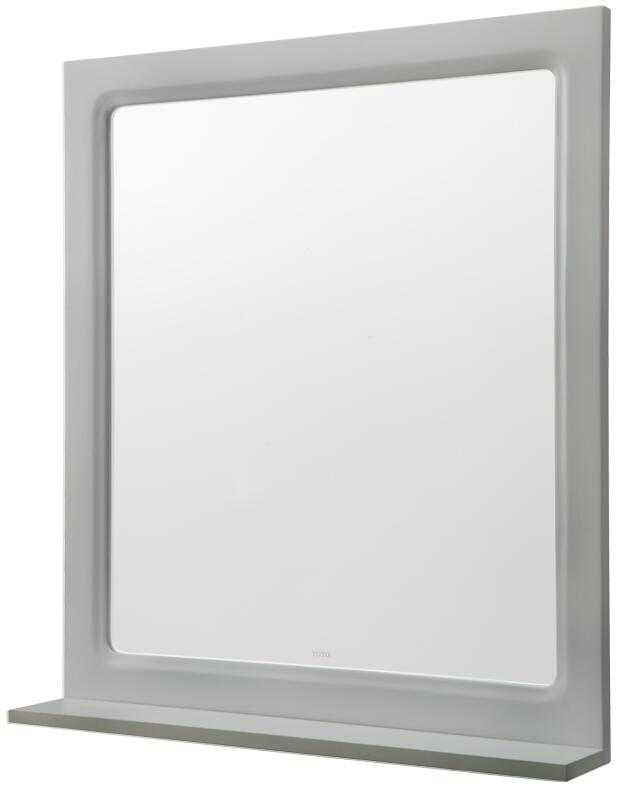13%
2020/10/1813%
2020/10/18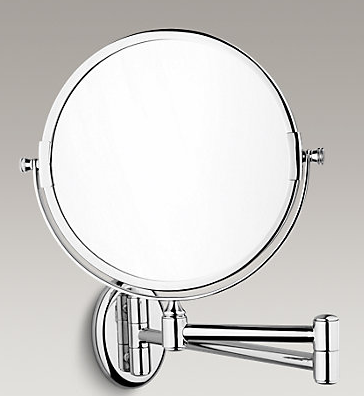13%
2020/10/18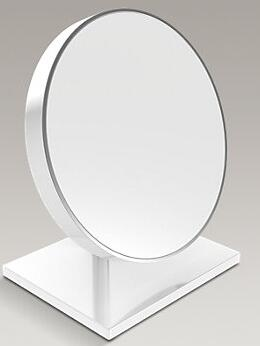13%
2020/10/18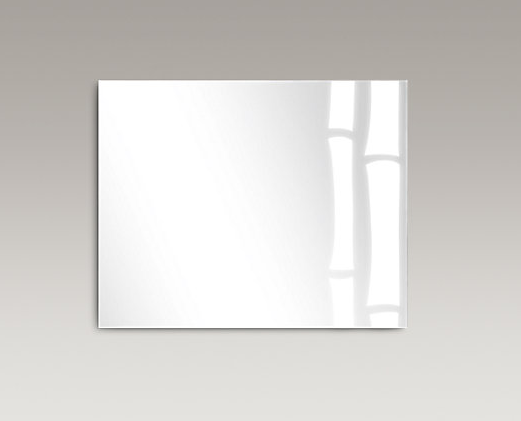13%
2020/10/18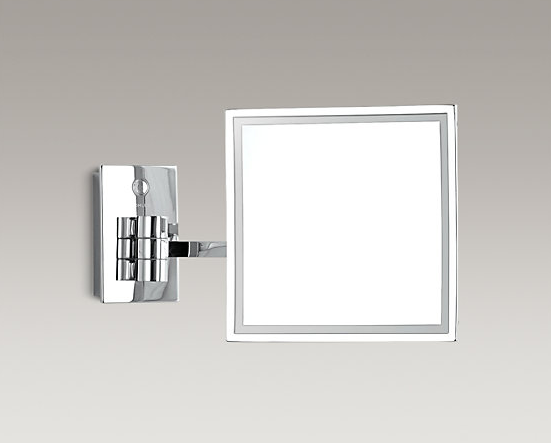13%
2020/10/18

• 1
• 2
• 3
• 4
• 5
• 6
• 62订阅中心广联达大数据